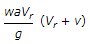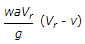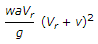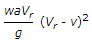# Mechanical Engineering - Hydraulic Machines

### Exercise :: Hydraulic Machines - Section 1

11.

The speed of a turbine runner is

 A. directly proportional to H1/2 B. inversely proportional to H1/2 C. directly proportional to H3/2 D. inversely proportional to H3/2

Explanation:

No answer description available for this question. Let us discuss.

12.

A ship with jet propulsion draws water through inlet orifices at right angles to the direction of its motion. The propelling force of the jet is (where a = Area of the jet, Vr = Relative velocity of the jet and ship = V + v, v = Velocity of the ship, and V = Velocity of the jet issuing from the ship)

 A.B.C.D.Explanation:

No answer description available for this question. Let us discuss.

13.

Discharge of a centrifugal pump is (where N = Speed of the pump impeller)

 A. directly proportional to N B. inversely proportional to N C. directly proportional to N2 D. inversely proportional to N2

Explanation:

No answer description available for this question. Let us discuss.

14.

In a reaction turbine, the draft tube is used

 A. to run the turbine full B. to prevent air to enter the turbine C. to increase the head of water by an amount equal to the height of the runner outlet above the tail race D. to transport water to downstream

Explanation:

No answer description available for this question. Let us discuss.

15.

The power produced by the reaction turbine is __________ to the head of water.

 A. directly proportional B. inversely proportional